# 分类问题–Logistic Regression方法介绍

• A+

## 例子引入

• 现在有两个Box, 里面装有一些三角（个数如下图所示）.
• 抽到Box1的概率为2/3，抽到Box2的概率为1/3.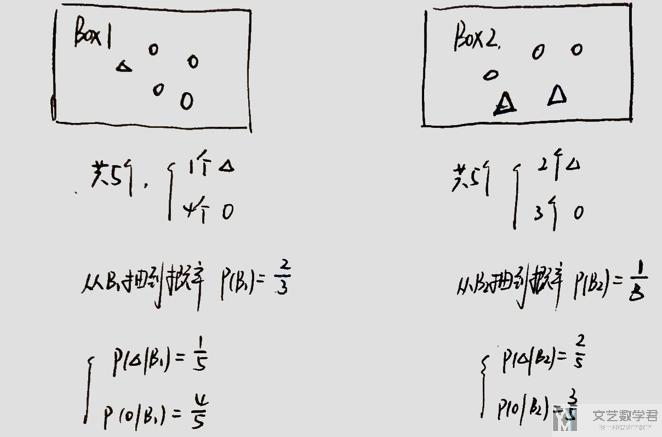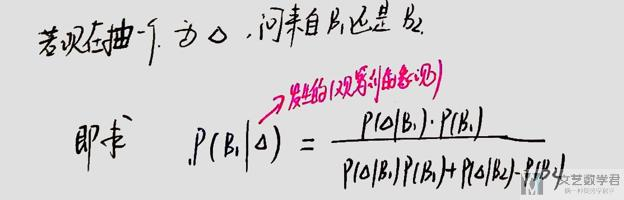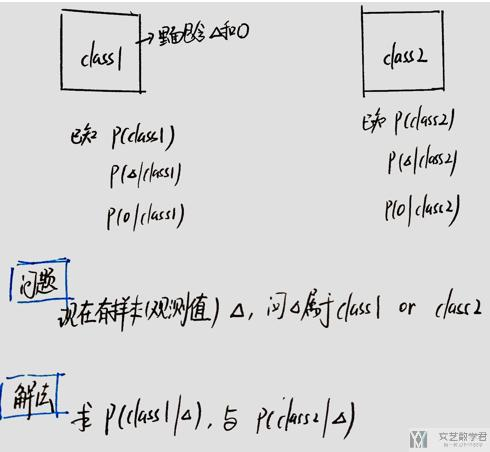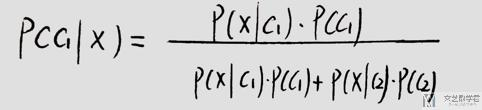## Generative Model

Generative Model的想法就是求出上面式子中四个概率的值。

### P(c1)和P(c2)的计算

P(c1)和P(c2)的计算没什么难度，可以直接进行计算.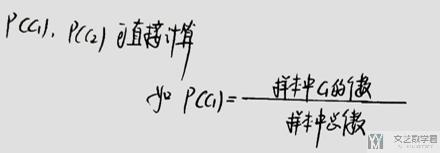### P(x|c1)和P(x|c2)的计算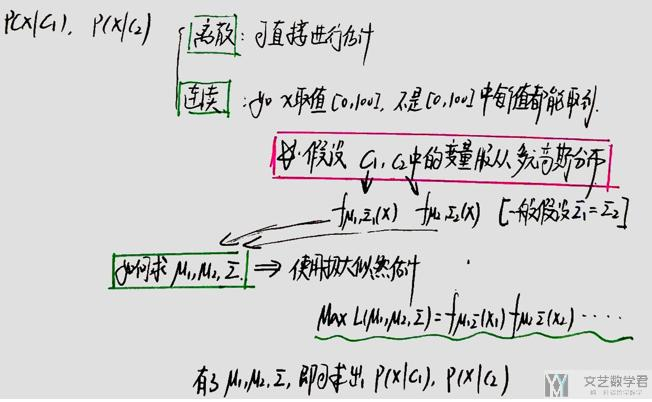## Logistic Model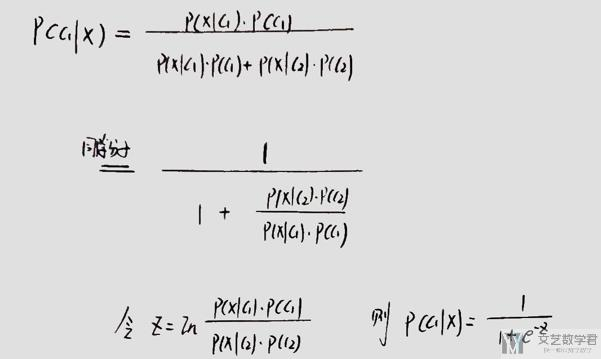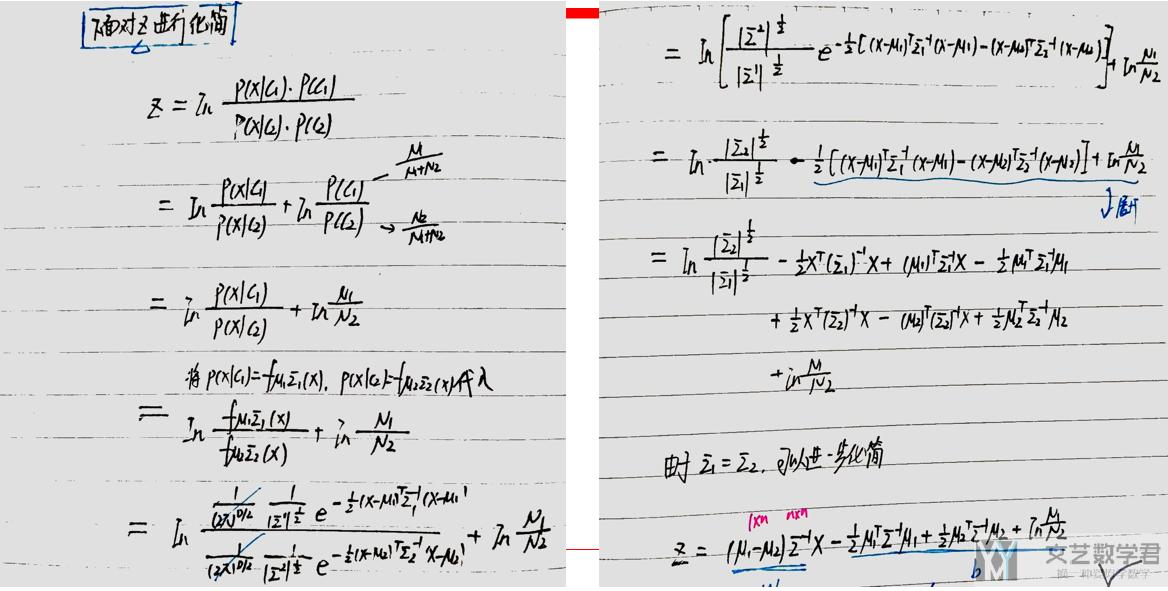### 求解w与b的值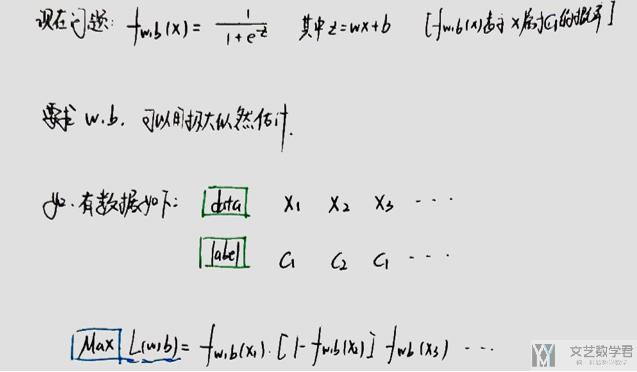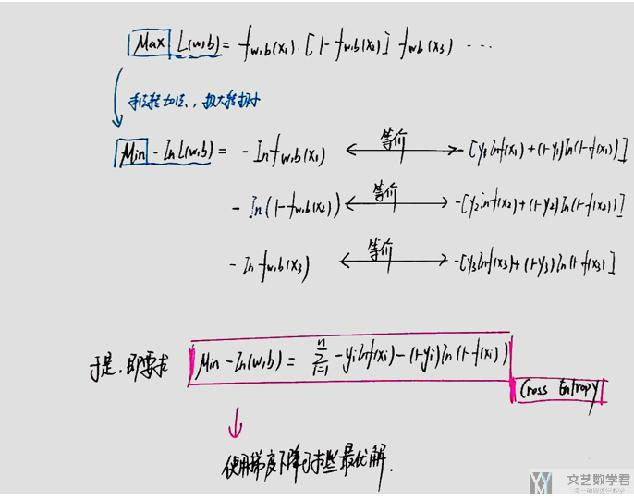• http://speech.ee.ntu.edu.tw/~tlkagk/courses_ML17_2.html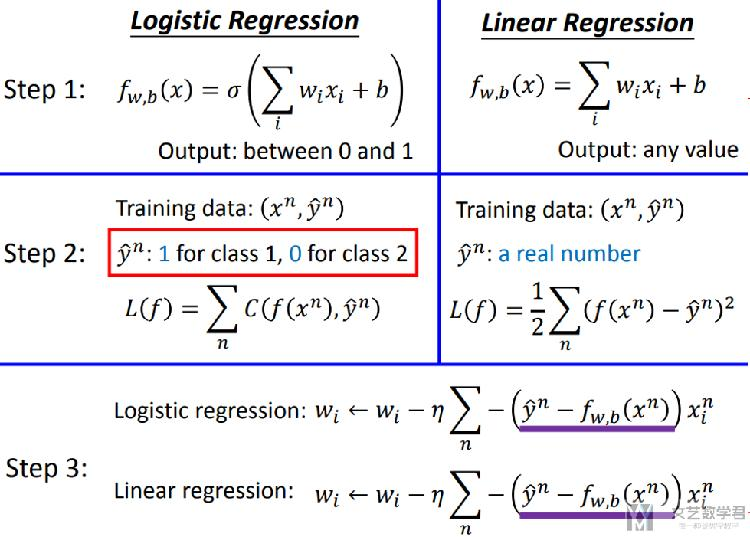## 多分类问题求解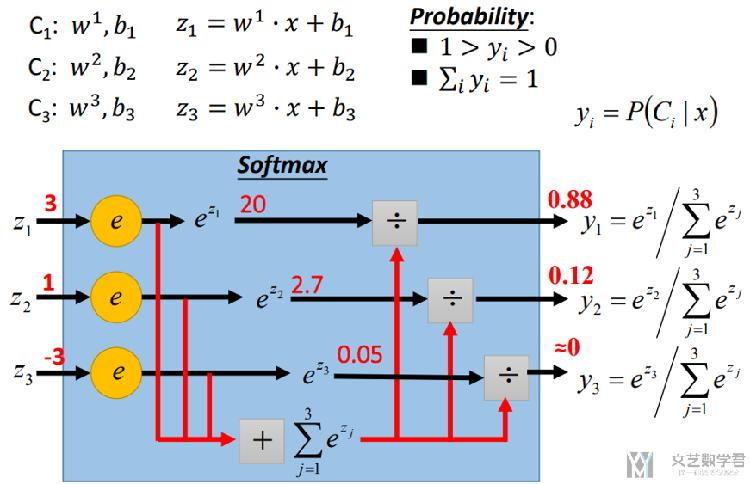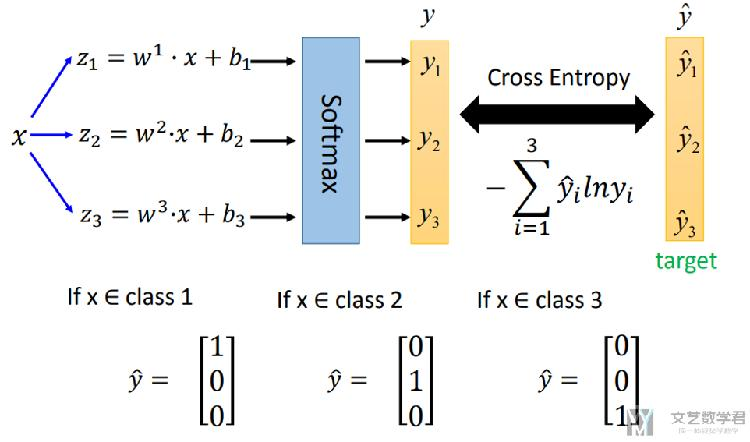### 二分类与多分类的类比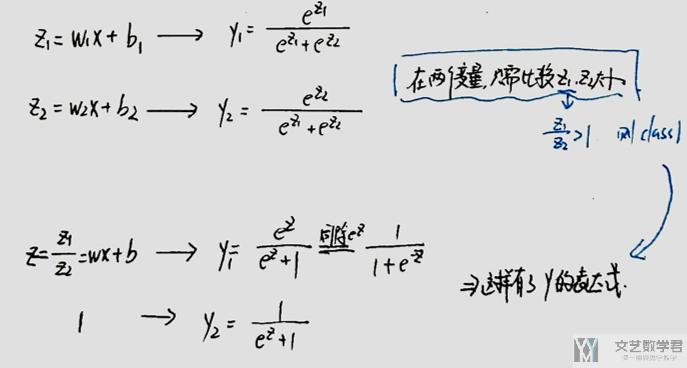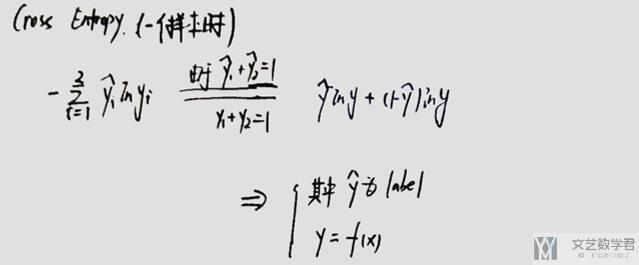## 逻辑回归实验

### 使用sklearn实现

1. from sklearn.linear_model import LogisticRegression
2. lr = LogisticRegression(solver='liblinear')
3. lr.fit(data.reshape(-1,1), labels.values.squeeze())#进行拟合
4. acc = lr.score(data.reshape(-1,1),labels.values.squeeze()) # 进行预测

### 使用PyTorch实现

1. class NeuralNet(nn.Module):
2.     def __init__(self, input_size, hidden_size, num_classes, n_layers):
3.         super(NeuralNet, self).__init__()
4.         self.LGLayer = nn.Linear(input_size, num_classes)
5.     def forward(self, x):
6.         out = self.LGLayer(x)
7.         return out
8. # CrossEntropyLoss里面就包含了softmax的步骤
9. criterion = nn.CrossEntropyLoss()

## 代码链接

GitHub仓库链接

• 微信公众号
• 关注微信公众号
•• QQ群
• 我们的QQ群号
•